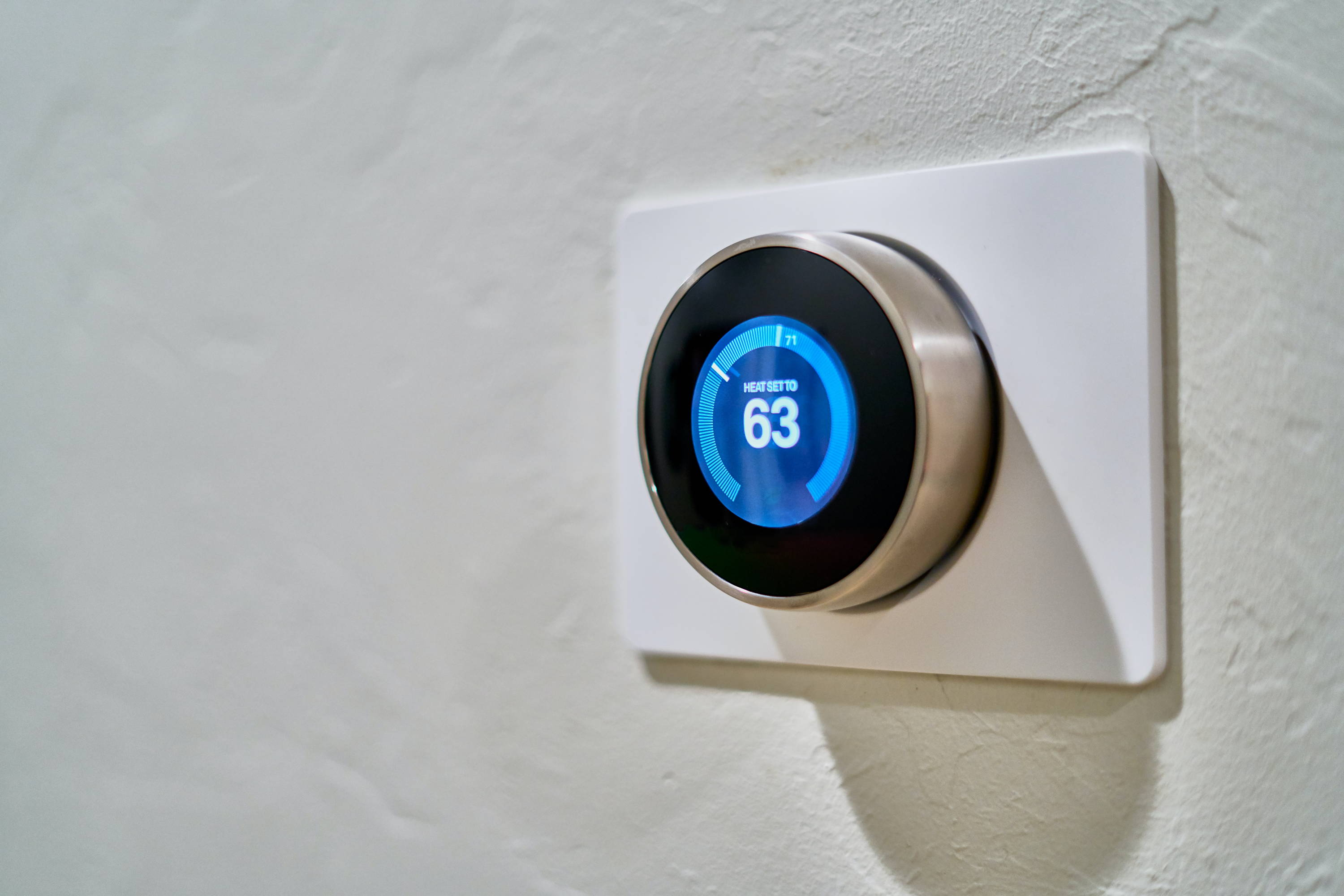Konnichi wa / Great Outdoors

## Introduction

This article will show you how to convert Celsius to Fahrenheit. To do this, you will need a calculator, paper and pencil. You can also use the conversion function on your phone if necessary.

Watch this video on a trick for converting Celsius to Fahrenheit!

## How to convert from Celsius to Fahrenheit:

Complete these two steps:

1. Multiply the Celsius temperature by 9/5 (or 1.8). For example, 180°C x 1.8 = 324.
2. Then, add 32 to the result of Step 1. So, ( 180°C x 1.8 ) + 32 = 356°F.

## How to convert from Fahrenheit to Celsius:

Complete these two steps (the above steps in reverse):

1. Subtract 32 from the Fahrenheit temperature. For example, 356°F - 32 = 324.
2. Multiply by 5/9 (or divide by 1.8). For example, 324 x 5/9 = 180 °C.

# The Great Outdoors Collection

Sold out
Sold out
Sold out
Sold out

## Temperature conversion table

 Fahrenheit (degrees F) Celsius (degrees C) Stage 32 degrees F 0 degrees C Freezing Point of Water 40 degrees F 4.4 degrees C 50 degrees F 10 degrees C 60 degrees F 15.6 degrees C 70 degrees F 21.1 degrees C 80 degrees F 26.7 degrees C 90 degrees F 32.2 degrees C 100 degrees F 37.8 degrees C 110 degrees F 43.3 degrees C 120 degrees F 48.9 degrees C 130 degrees F 54.4 degrees C 140 degrees F 60 degrees C 150 degrees F 65.6 degrees C 160 degrees F 71.1 degrees C 170 degrees F 76.7 degrees C 180 degrees F 82.2 degrees C 190 degrees F 87.8 degrees C 200 degrees F 93.3 degrees C 212 degrees F 100 degrees C Boiling Point of Water 220 degrees F 104 degrees C 230 degrees F 110 degrees C 240 degrees F 115 degrees C 250 degrees F 121 degrees C 260 degrees F 127 degrees C 270 degrees F 132 degrees C 280 degrees F 138 degrees C 290 degrees F 143 degrees C 300 degrees F 149 degrees C 320 degrees F 160 degrees C 340 degrees F 170 degrees C 350 degrees F 177 degrees C 400 degrees F 205 degrees C 450 degrees F 233 degrees C 500 degrees F 260 degrees C

## Final thoughts on 180 C to F

“The mountains are calling and I must go.”

— John Muir